# NCERT SOLUTION FOR CLASS 8 MATHS CHAPTER 3 UNDERSTANDING QUADRILATERALS

The National Council of Educational Research and Training (NCERT) is an autonomous body of the Indian government that formulates the curricula for schools in India that are governed by the Central Board of Secondary Education (CBSE) and certain state boards. Therefore, students who will be taking the Class 10 tests administered by various boards should consult this NCERT Syllabus in order to prepare for those examinations, which in turn will assist those students get a passing score.

You can also download the NCERT SOLUTION FOR CLASS 8 MATHS CHAPTER 3 UNDERSTANDING QUADRILATERALS to assist you in comprehensively reviewing the course material and achieving a higher score on your upcoming assessments.

Students are able to create a strong conceptual basis with the help of the NCERT Solutions PDFs available at SWC. This base plays a key part in the students’ preparation for the later phases of the competitive examinations. We give comprehensive answers to challenges posed by NCERT in accordance with the methodology provided by CBSE. The students are able to effortlessly prepare all of the ideas that are presented in their respective courses better and more successfully, and they are even able to succeed in passing the most difficult competitive examinations, such as JEE Main, JEE Advanced, NEET, AIMS, and so on.

## NCERT Solutions for Class 8 Maths Chapter 3 – Exercises

NCERT Solutions for Class 8 Maths Exercise 3.1

Question 1.
Given here are some figures: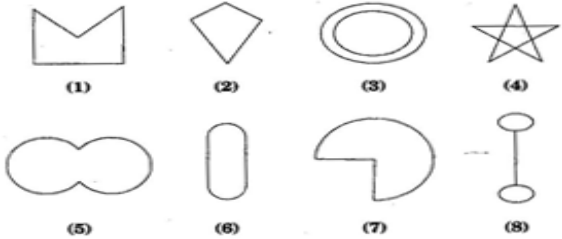Classify each of them on the basis of the following:
(a) Simple curve (b) Simple closed curve (c) Polygon (d) Convex polygon (e) Concave polygon

Solution :
(a) Simple curve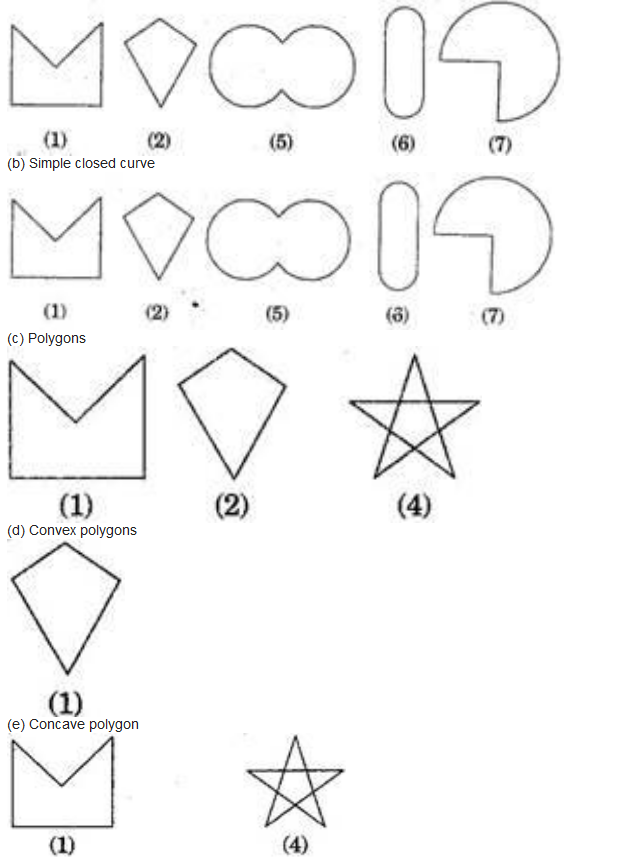(b) Simple closed curve

Question 2.
How many diagonals does each of the following have?
(b) A regular hexagon
(c) A triangle
Solution :
(a) A convex quadrilateral has two diagonals.
Here, AC and BD are two diagonals.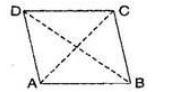(b) A regular hexagon has 9 diagonals.
Here, diagonals are AD, AE, BD, BE, FC, FB, AC, EC and FD.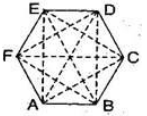(c) A triangle has no diagonal.
Question 3.
What is the sum of the measures of the angles of a convex quadrilateral? Will this property hold if the quadrilateral is not convex? (Make a non-convex quadrilateral and try)
Solution :
Let ABCD is a convex quadrilateral, then we draw a diagonal AC which divides the quadrilateral in two triangles.
∠A + ∠B + ∠C + ∠D
= ∠1 + ∠6 + ∠5 + ∠4 + ∠3 + ∠2
= (∠1 + ∠2 + ∠3) + (∠4 + ∠5 + ∠6)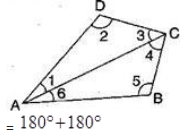[By Angle sum property of triangle]
360º
Hence, the sum of measures of the triangles of a convex quadrilateral is 360º
Yes, if quadrilateral is not convex then, this property will also be applied.
Let ABCD is a non-convex quadrilateral and join BD, which also divides the quadrilateral in two triangles.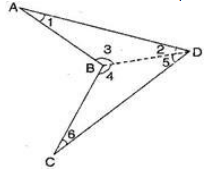Using angle sum property of triangle,
In △ABD, ∠1 + ∠2 + ∠3 = 180º;……….(i)
In △BDC, ∠4 + ∠5 + ∠6 = 180º;……….(i)
∠1 + ∠2 + ∠3 + ∠4 + ∠5 + ∠6 = 360º
⇒;∠1 + ∠2 + (∠3 + ∠4) + ∠5 + ∠6
= 360º
⇒;∠A + ∠B + ∠C + ∠D = 360º
Hence proved.
Question 4.
Examine the table. (Each figure is divided into triangles and the sum of the angles deduced from that.)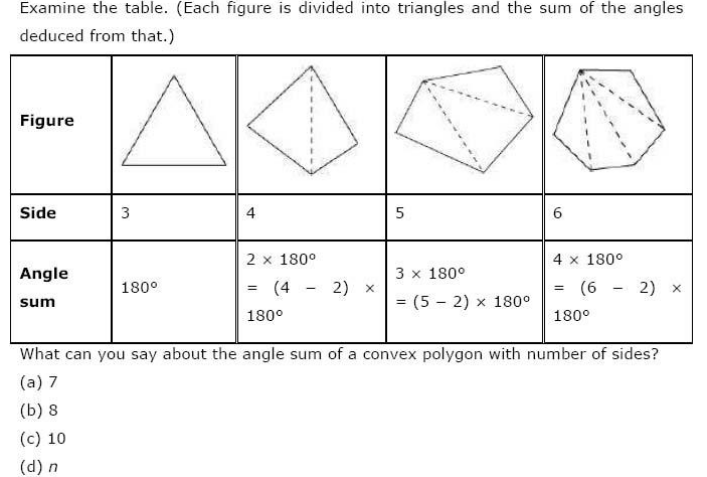Solution :
(a) When n = 7, then
Angle sum of a polygon =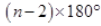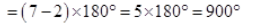(b) When n= 8, then
Angle sum of a polygon =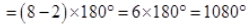(c) When n = 10, then
Angle sum of a polygon =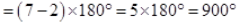(d) When n = n then
Angle sum of a polygon =Question 5.
What is a regular polygon? State the name of a regular polygon of:
(a) 3 sides
(b) 4 sides
(c) 6 sides
Solution :
A regular polygon: A polygon having all sides of equal length and the interior angles of equal size is known as regular polygon.
(i) 3 sides
Polygon having three sides is called a triangle.
(ii) 4 sides
Polygon having four sides is called a quadrilateral.
(iii) 6 sides
Polygon having six sides is called a hexagon.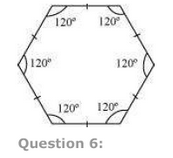x = 140º

(d)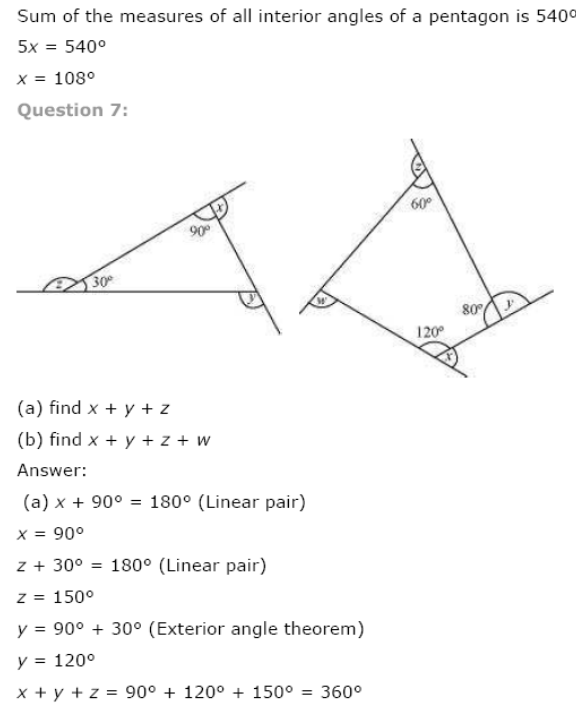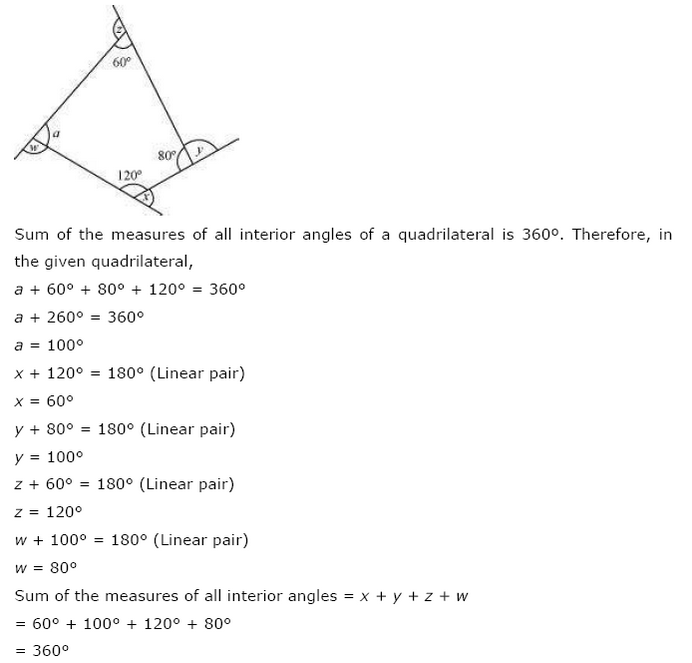NCERT Solutions for Class 8 Maths Exercise 3.2

Question 1.
Find x;in the following figures: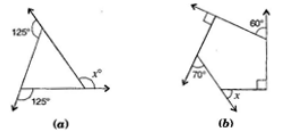Solution :
(a) Here,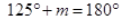[Linear pair]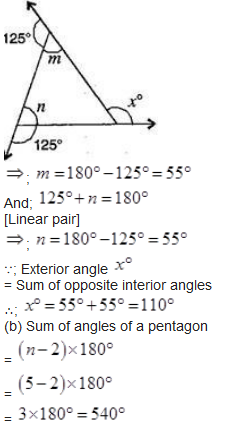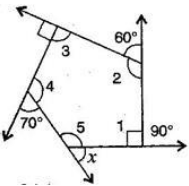By linear pairs of angles,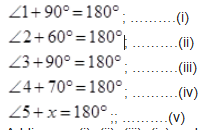Adding eq. (i), (ii), (iii), (iv) and (v),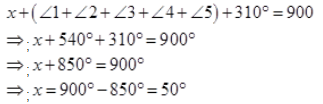Question 2.
Find the measure of each exterior angle of a regular polygon of:
(a) 9 sides
(b) 15 sides
Solution :
(i) Sum of angles of a regular polygon =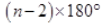=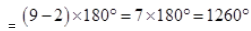Each interior angle =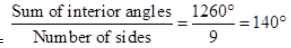Each exterior angle =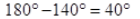(ii) Sum of exterior angles of a regular polygon = 360º
Each interior angle =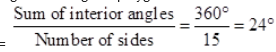Question 3.
How many sides does a regular polygon have, if the measure of an exterior angle is 24º?

Solution :
Let number of sides be  n.
Sum of exterior angles of a regular polygon = 360º
Number of sides =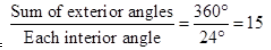Hence, the regular polygon has 15 sides.

Question 4.
How many sides does a regular polygon have if each of its interior angles is  165º?
Solution :
Let number of sides be n.
Exterior angle =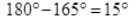Sum of exterior angles of a regular polygon = 360º
Number of sides =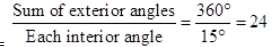Hence, the regular polygon has 24 sides.
Question 5.
(a) Is it possible to have a regular polygon with of each exterior angle as 22º?
(b) Can it be an interior angle of a regular polygon? Why?
Solution :
(a) No. (Since 22 is not a divisor of 360º)
(b) No, (Because each exterior angle is 180º –  22º = 158º; which is not a divisor of 360º)

Question 6.

(a) What is the minimum interior angle possible for a regular polygon? Why?
(b) What is the maximum exterior angle possible for a regular polygon?
Solution :
(a) The equilateral triangle being a regular polygon of 3 sides has the least measure of an
interior angle of 60º,
∵ Sum of all the angles of a triangle
180º
∴;   x + x +x = 180º
⇒     3 x = 180º
⇒       x  = 60º
(b) By (a), we can observe that the greatest exterior angle is   180º –  60º
= 120º.

NCERT Solutions for Class 8 Maths Exercise 3.3

Question 1.
Given a parallelogram ABCD. Complete each statement along with the definition or property used.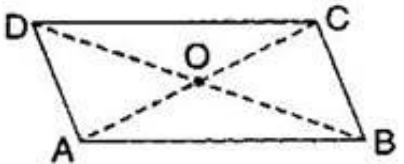(ii) ∠DCB = ______________
(iii) OC = _____________
(iv) m∠DAB + m∠CDA = ________

Solution :
[Since opposite sides of a parallelogram are equal]
(ii) ∠DCB = ∠DAB
[Since opposite angles of a parallelogram are equal]
(iii) OC = OA
[Since diagonals of a parallelogram bisect each other]
(iv) m∠DAB + m∠CDA = 180º
[Adjacent angles in a parallelogram are supplementary]

Question 2.
Consider the following parallelograms. Find the values of the unknowns x , y ,z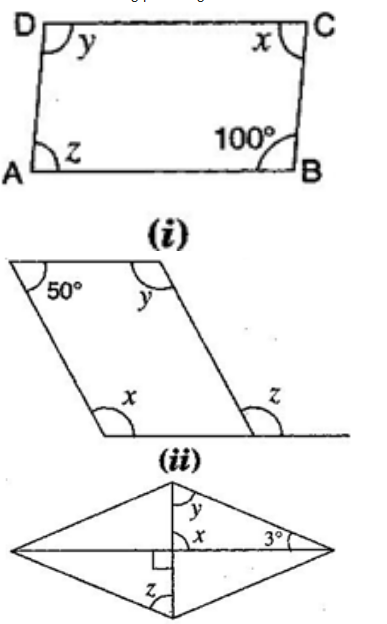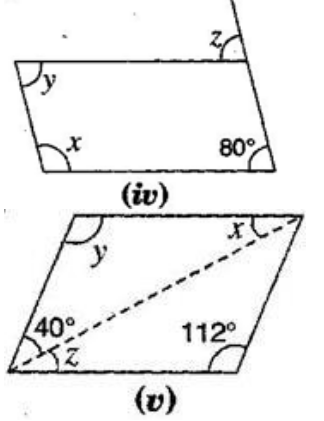Note: For getting correct answer, read  3º  =  30º in figure (iii)
Solution :
(i) ∠B + ∠C = 180º

[Adjacent angles in a parallelogram are supplementary]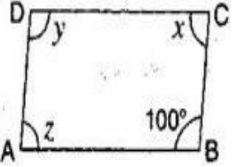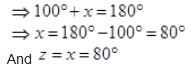[Since opposite angles of a parallelogram are equal]
Also   y = 100º
[Since opposite angles of a parallelogram are equal]
(ii)  x + 50º = 180º x = 50º = 180º
[Adjacent angles in a ||gm are supplementary]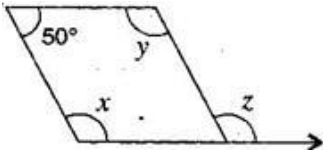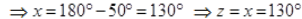[Corresponding angles]
(iii)  x = 90º
[Vertically opposite angles]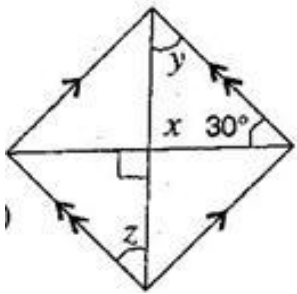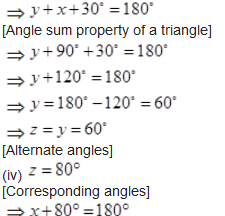[Adjacent angles in a ||gm are supplementary]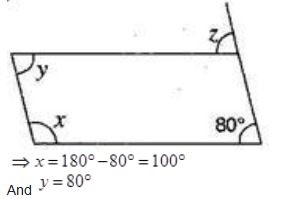[Opposite angles are equal in a ||gm]
(v)  y = 112º
[Opposite angles are equal in a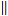gm]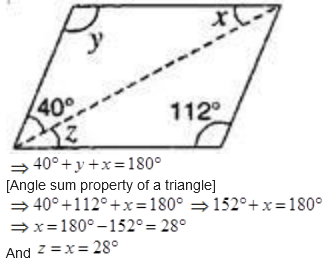[Alternate angles]

Question 3.

Can a quadrilateral ABCD be a parallelogram, if:
(i) ∠D + ∠B = 180º
(ii) AB = DC = 8 cm, AD = 4 cm and BC = 4.4 cm?
(iii) ∠A = 70º and ∠C = 65º?

Solution :
(i) ∠D + ∠B = 180º
It can be, but here, it needs not to be.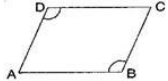(ii) No, in this case because one pair of opposite sides are equal and another pair of opposite sides are unequal. So, it is not a parallelogram.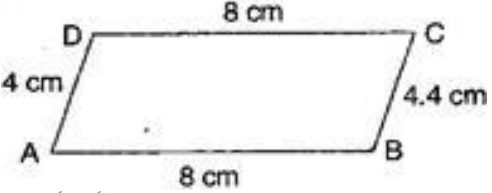(iii) No.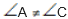Since opposite angles are equal in parallelogram and here opposite angles are not equal in quadrilateral ABCD. Therefore it is not a parallelogram.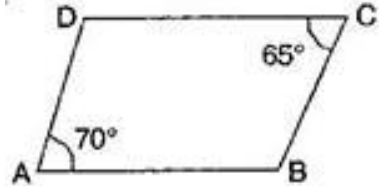Question 4.

Draw a rough figure of a quadrilateral that is not a parallelogram but has exactly two opposite angles of equal measures.

Solution :
ABCD is a quadrilateral in which angles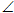A =C = 110º
Therefore, it could be a kite.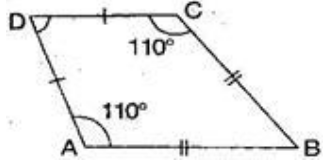Question 5.

The measure of two adjacent angles of a parallelogram are in the ratio 3 : 2. Find the measure of each of the angles of the parallelogram.

Solution :
Let two adjacent angles be 3X and 2X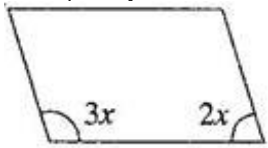Since the adjacent angles in a parallelogram are supplementary.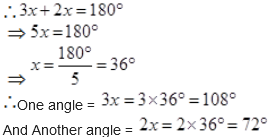Question 6.

Two adjacent angles of a parallelogram have equal measure. Find the measure of the angles of the parallelogram.

Solution : Let each adjacent angle be x,
Since the adjacent angles in a parallelogram are supplementary.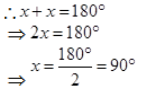Hence, each adjacent angle is 90º

Question 7.
The adjacent figure HOPW is a parallelogram. Find the angle measures  x , y and z State the properties you use to find them.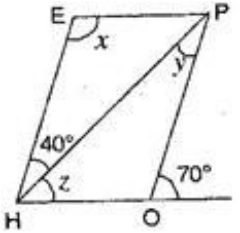∠HOP +  70º = 180º

Question 8.
The following figures GUNS and RUNS are parallelograms. Find x and (Lengths are in cm)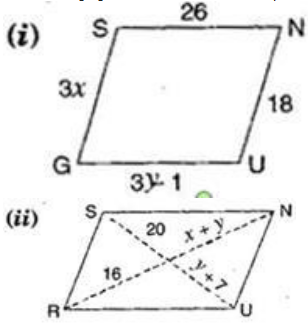Solution :

(i) In parallelogram GUNS,
GS = UN
[Opposite sides of parallelogram are equal]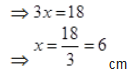Also GU = SN
[Opposite sides of parallelogram are equal]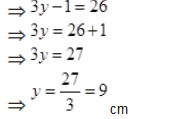Hence, x= 6 cm and x= 9 cm.
(ii) In parallelogram RUNS,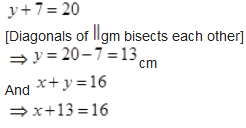⇒ x = 16 -13

⇒ x = 16 -13

⇒ x = 3cm

Hence,  x = 3 cm and y = 13cm.

Question 9.
In the figure, both RISK and CLUE are parallelograms. Find the value of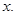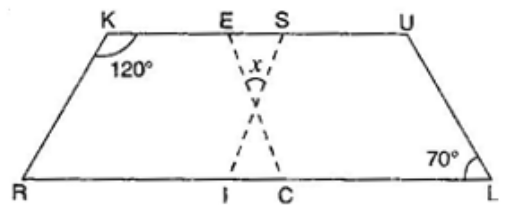Solution :

In parallelogram RISK,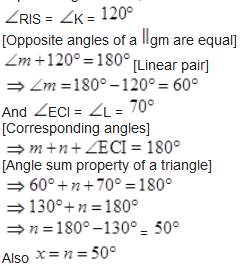[Vertically opposite angles]

Question 10.

Explain how this figure is a trapezium. Which is its two sides are parallel?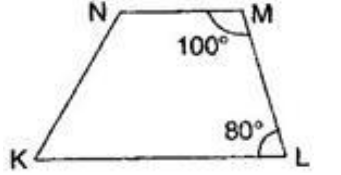Solution :
Here, ∠M + ∠L =  100º + 80º = 180º
[Sum of interior opposite angles is 180º]
NM and KL are parallel.
Hence, KLMN is a trapezium.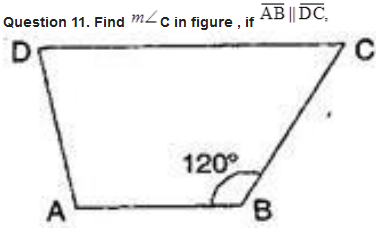Solution :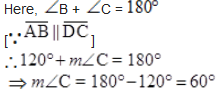Question 12.
Find the measure of ∠P and ∠S if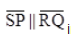in given figure.
(If you find m∠R is there more than one method to find m∠P)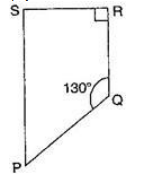Solution :

NCERT Solutions for Class 8 Maths Exercise 3.4

## Conclusions for NCERT Solutions of Class 8 Maths Chapter 3

The academic team of SWC has provided NCERT answers for class 8 mathematics chapter-3. We’ve given solutions for all of Chapter 3’s exercises. These are step-by-step answers to all of the problems in Chapter 3 of the NCERT textbook. Read the first theoretical chapter. Make sure you’ve gone through the theoretical section of chapter 2 3in the NCERT textbook and that you know the formula for the chapter.

Your academic journey begins a new stage as you reach the eighth grade, and a new facet of learning awaits you. This is the year where pupils begin to acquire an interest in the topic at hand, and it is also the year in which you choose whether you will study business, humanity, or science in the following year (class 12). As a consequence of this, having a solid foundation of knowledge in fields such as mathematics and physics comes highly recommended. The NCERT textbook for class 8 is the greatest book now available on the market for enhancing one’s education in the areas of mathematics and science. Read up on the theory that is presented in the book for grade 8 and answer all of the questions that are posed in the practice section of the NCERT textbook.

When working through the exercises in the NCERT textbook, if you run into any type of difficulty or uncertainty, you may use the swc NCERT Solutions for class 8 as a point of reference. While you are reading the theory form textbook, it is imperative that you always have notes prepared. You should make an effort to understand things from the very beginning so that you may create a solid foundation in the topic. Use the NCERT as your parent book to ensure that you have a strong foundation. After you have finished reading the theoretical section of the textbook, you should go to additional reference books.